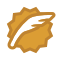# 刷题一个4ms的程序，代码如何优化到3ms再到2ms？

## 前言## 具体

public int maxArea2(int[] height) {
int max = 0;
int left = 0;
int right = height.length - 1;
while (left < right) {
max = Math.max(max, Math.min(height[left], height[right]) * (right - left));
if (height[left] > height[right])// 右侧更小
right--;
else {
left++;
}
}
return max;
}• 使用Math.max()判断最大值最小值的时候，下面在判断是左指针右移还是右指针左移动重复判断了，我们可以手动比较大小重复利用这次计算去完成相同的操作。
public int maxArea3(int[] height) {
int max = 0;
int left = 0;
int right = height.length - 1;
while (left < right) {
if (height[left] < height[right]) {
max = Math.max(max, height[left] * (right - left));
left++;
} else {
max = Math.max(max, height[left] * (right - left));
right--;
}
}
return max;
}• Math.max()返回double类型转成int需要一定成本，然而我们数据本身就是int类型可以直接计算。
• 对数组取值的时候，比较取一次(两个值)，计算取一次(一个值)，而我们知道数组其实在内存中我们通过0号位置计算得到我们对应位置的数值，所以我们可以把3次计算减少成2次，用两个空间leftvalue和rightvalue记录左右数组位置的值。

public int maxArea4(int[] height) {
int max = 0;
int left = 0;
int right = height.length - 1;
int team = 0;
int leftvalue=0;int rightvalue=0;
while (left < right)  {
leftvalue=height[left];rightvalue=height[right];
if (leftvalue < rightvalue) {
team = leftvalue * (right-left);
if(max<team) {max=team;}
left++;
} else {
team = rightvalue * (right-left);
if(max<team) {max=team;}
right--;
}
}
return max;
}• 我是暂时不能了，，各位大佬请便！

## 结语

Big saiCSDN认证博客专家 scikit-learn

01-3081609-201581
12-17441
03-262387
12-14450
09-27809
01-23460
05-06515
10-163581
04-30118